# RD Sharma Solutions for Class 9 Maths Chapter 10 Congruent Triangles Exercise 10.1

Exercise 10.1 of RD Sharma textbook is based on the topic of congruent triangles and students will need to solve problems based on this topic. However, to just give you a gist, a triangle is a three-sided polygon having three angles which sum up to 1800. When it comes to congruence of triangles, two triangles are congruent if they superimpose on each other or if their sides and angles have the same lengths and measurements respectively. Check the detailed RD Sharma solutions for class 9 chapter 10 congruent triangles below.

## Download PDF of RD Sharma Solutions for Class 9 Maths Chapter 10 Congruent Triangles Exercise 10.1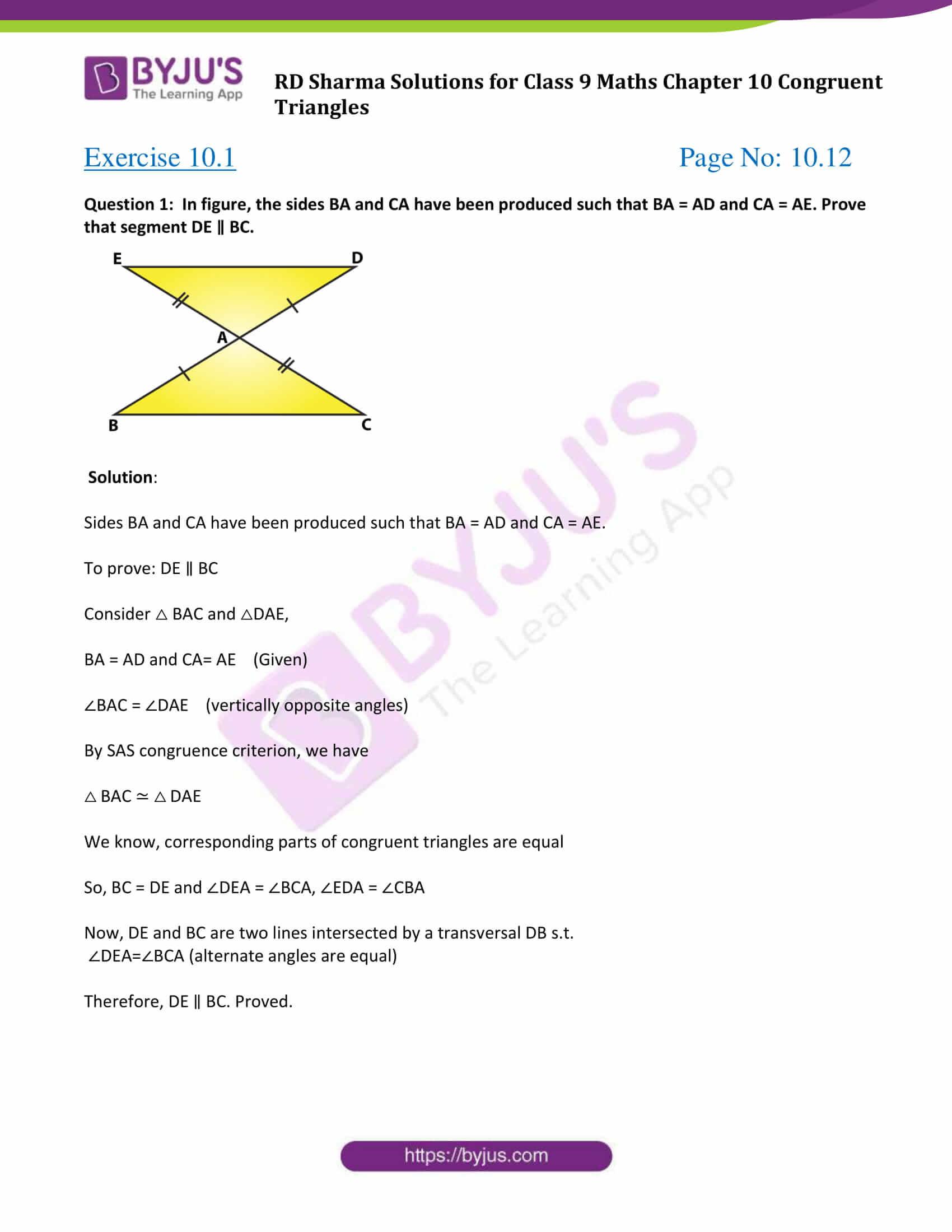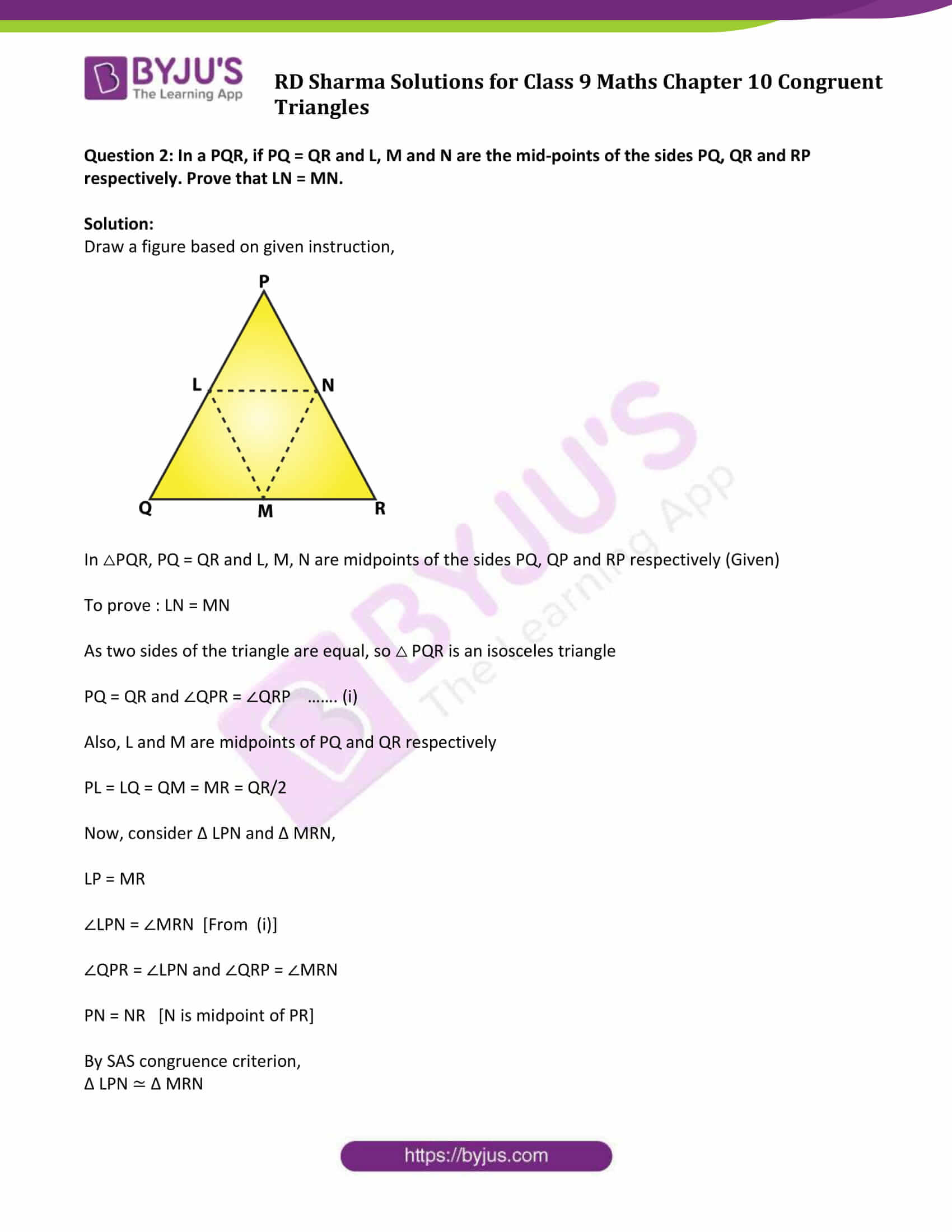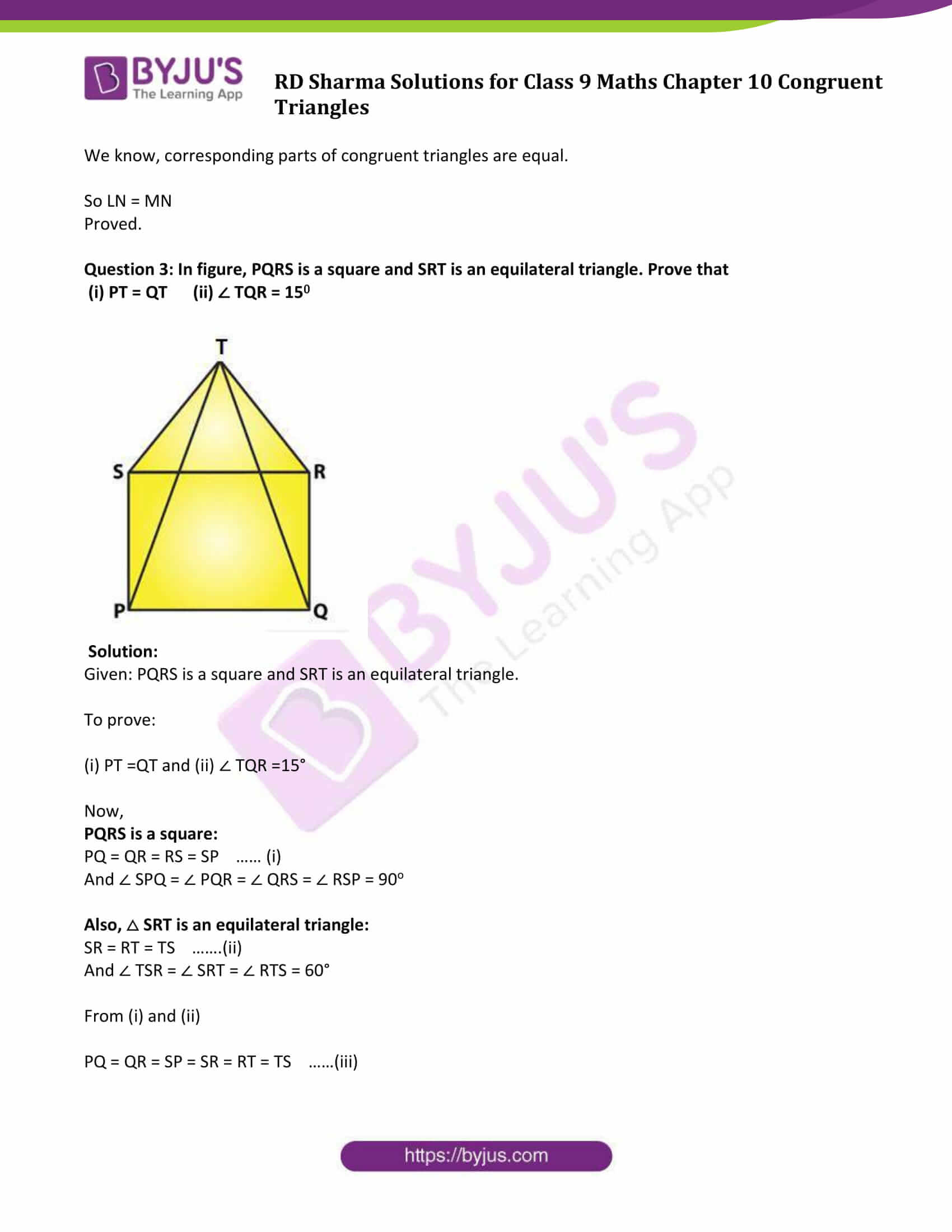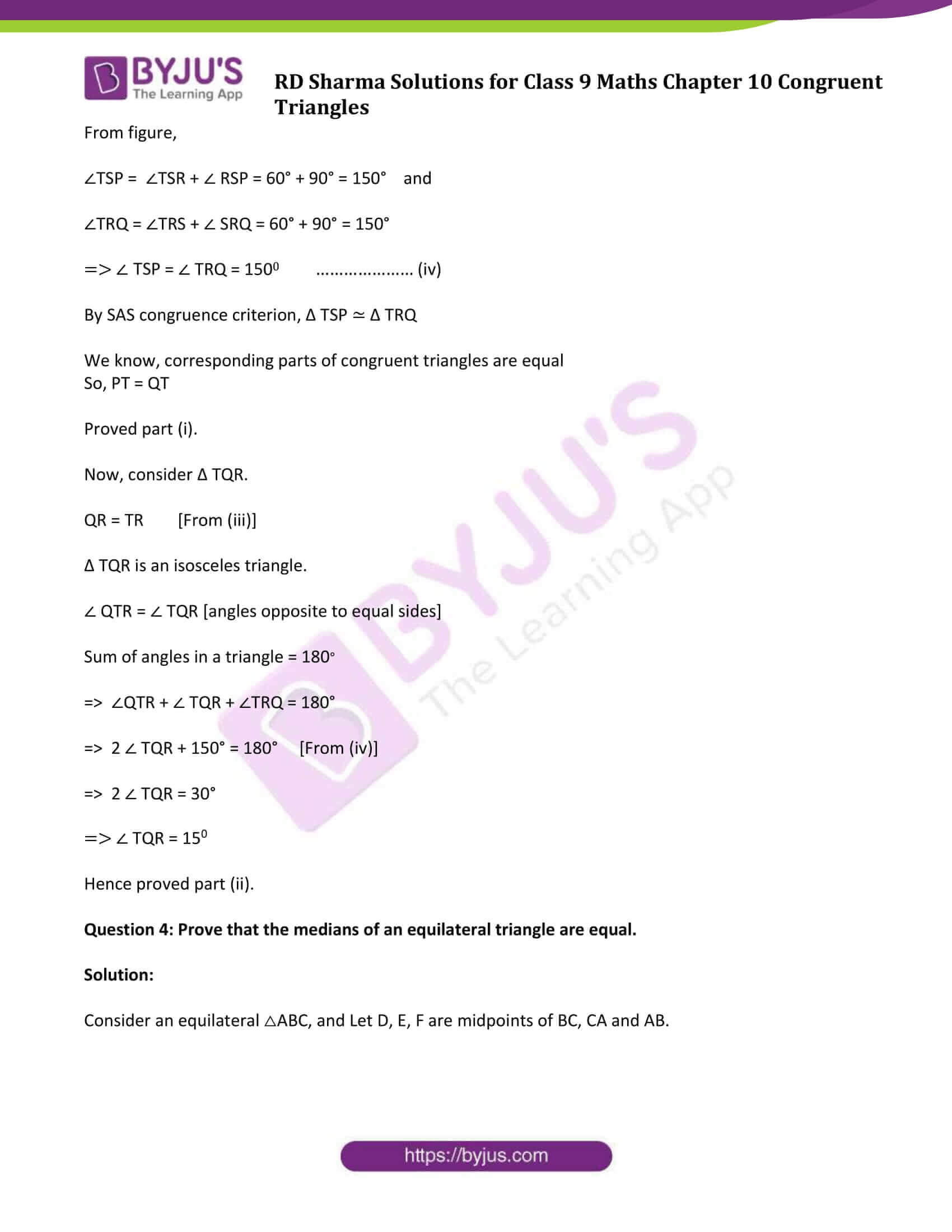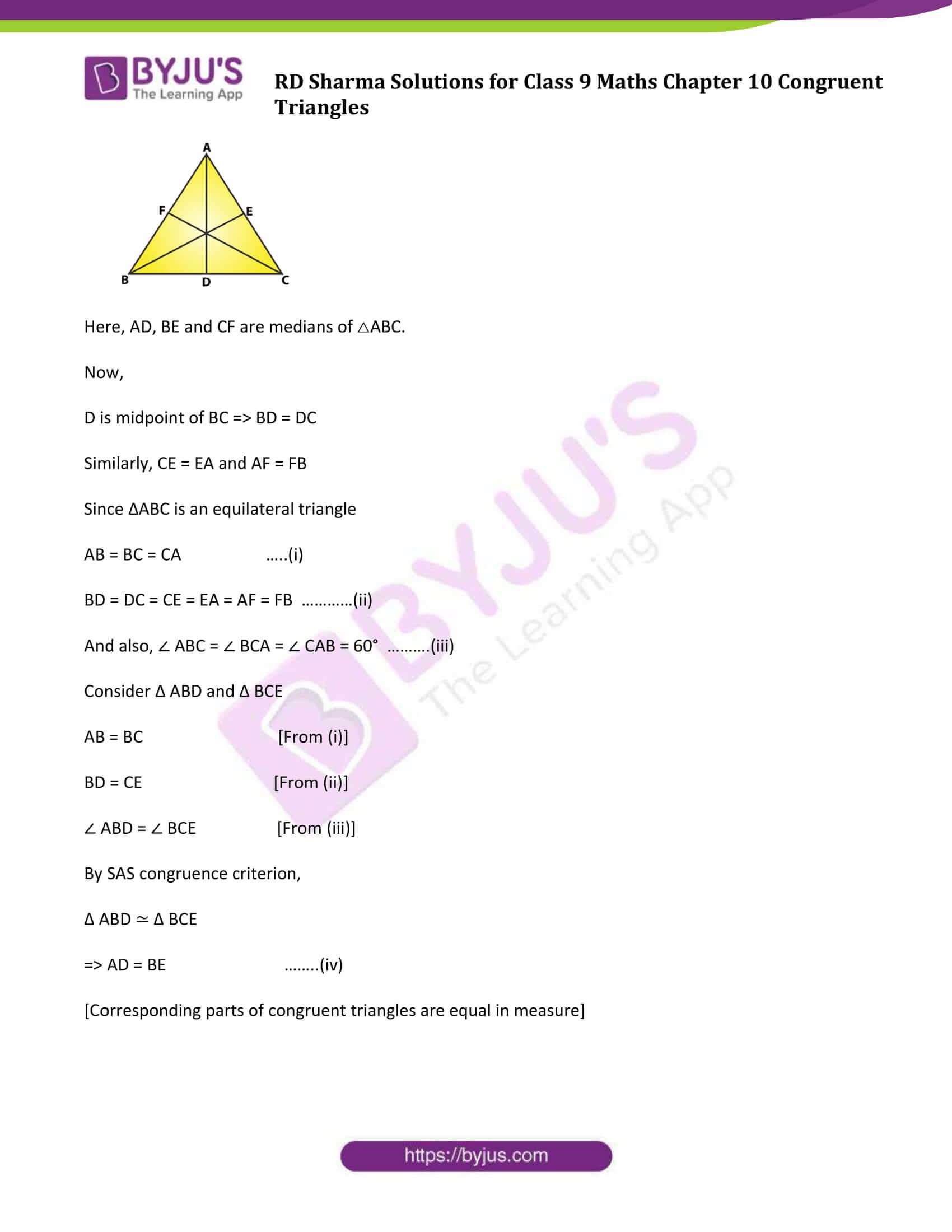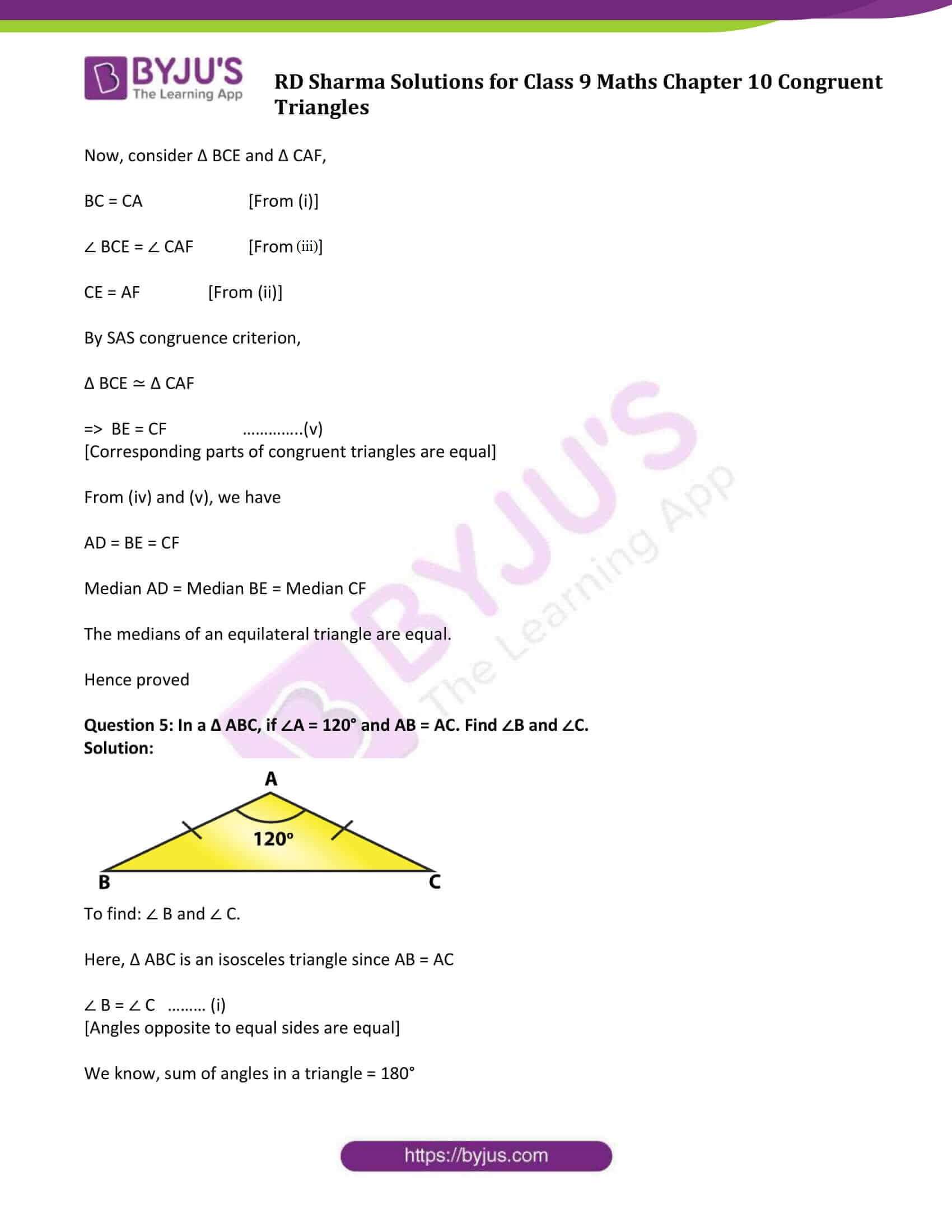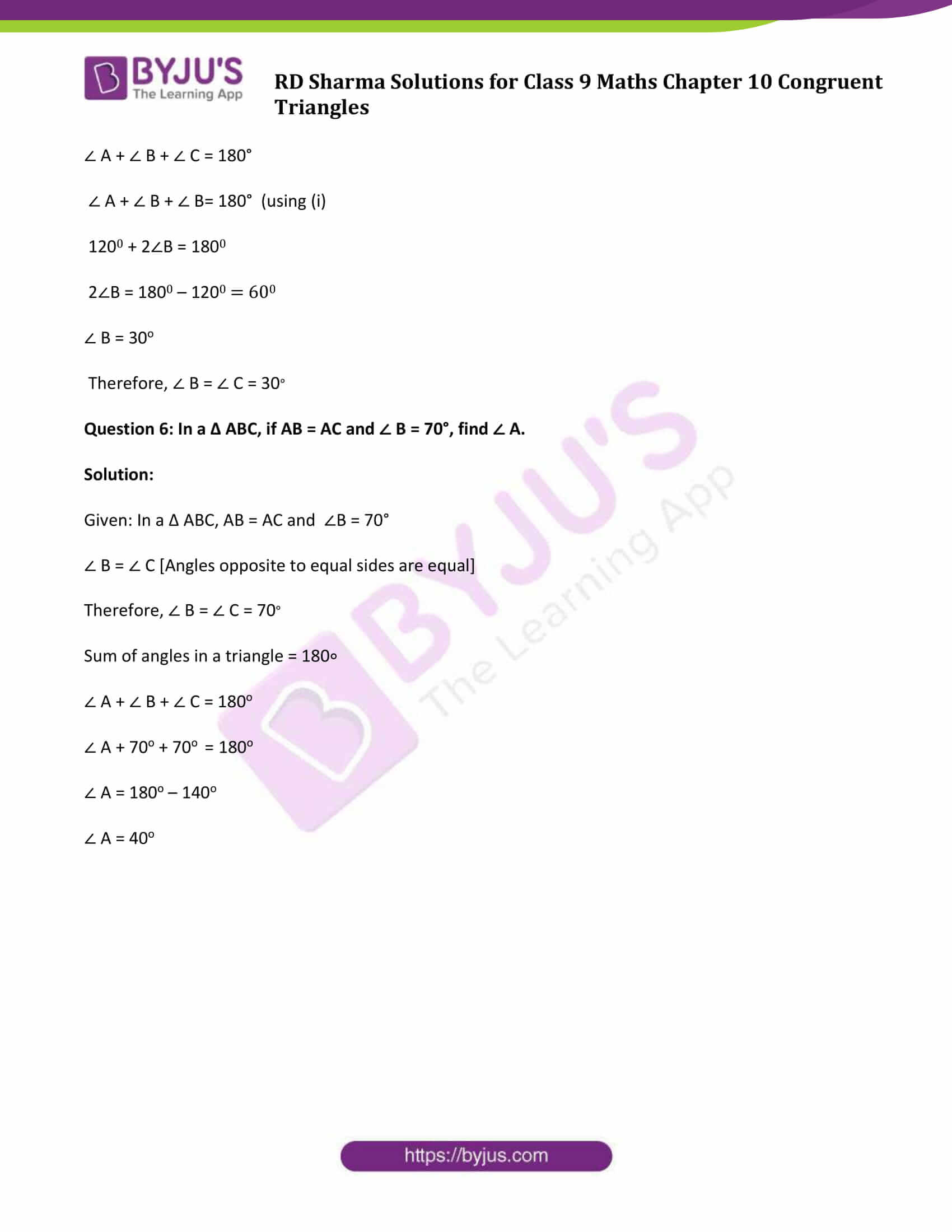### Access Answers to Maths RD Sharma Solutions for Class 9 Chapter 10 Congruent Triangles Exercise 10.1 Page number 10.12

Question 1: In figure, the sides BA and CA have been produced such that BA = AD and CA = AE. Prove that segment DE ∥ BC.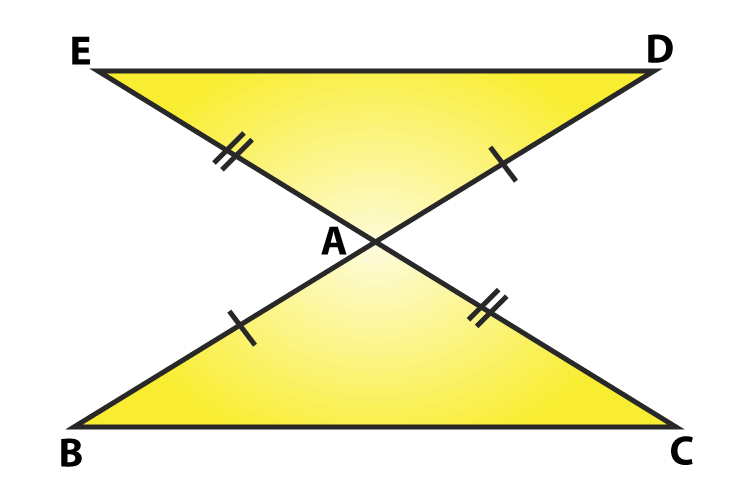Solution:

Sides BA and CA have been produced such that BA = AD and CA = AE.

To prove: DE ∥ BC

Consider △ BAC and △DAE,

BA = AD and CA= AE (Given)

∠BAC = ∠DAE (vertically opposite angles)

By SAS congruence criterion, we have

△ BAC ≃ △ DAE

We know, corresponding parts of congruent triangles are equal

So, BC = DE and ∠DEA = ∠BCA, ∠EDA = ∠CBA

Now, DE and BC are two lines intersected by a transversal DB s.t.

∠DEA=∠BCA (alternate angles are equal)

Therefore, DE ∥ BC. Proved.

Question 2: In a PQR, if PQ = QR and L, M and N are the mid-points of the sides PQ, QR and RP respectively. Prove that LN = MN.

Solution:

Draw a figure based on given instruction,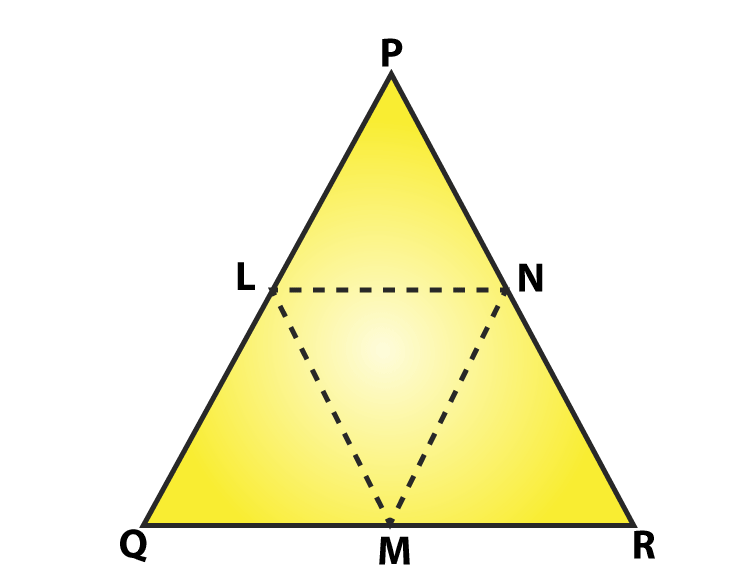In △PQR, PQ = QR and L, M, N are midpoints of the sides PQ, QP and RP respectively (Given)

To prove : LN = MN

As two sides of the triangle are equal, so △ PQR is an isosceles triangle

PQ = QR and ∠QPR = ∠QRP ……. (i)

Also, L and M are midpoints of PQ and QR respectively

PL = LQ = QM = MR = QR/2

Now, consider Δ LPN and Δ MRN,

LP = MR

∠LPN = ∠MRN [From (i)]

∠QPR = ∠LPN and ∠QRP = ∠MRN

PN = NR [N is midpoint of PR]

By SAS congruence criterion,

Δ LPN ≃ Δ MRN

We know, corresponding parts of congruent triangles are equal.

So LN = MN

Proved.

Question 3: In figure, PQRS is a square and SRT is an equilateral triangle. Prove that

(i) PT = QT (ii) ∠ TQR = 150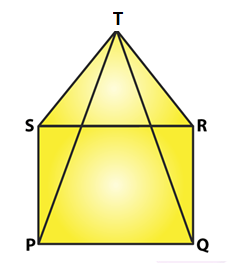Solution:

Given: PQRS is a square and SRT is an equilateral triangle.

To prove:

(i) PT =QT and (ii) ∠ TQR =15°

Now,

PQRS is a square:

PQ = QR = RS = SP …… (i)

And ∠ SPQ = ∠ PQR = ∠ QRS = ∠ RSP = 90o

Also, △ SRT is an equilateral triangle:

SR = RT = TS …….(ii)

And ∠ TSR = ∠ SRT = ∠ RTS = 60°

From (i) and (ii)

PQ = QR = SP = SR = RT = TS ……(iii)

From figure,

∠TSP = ∠TSR + ∠ RSP = 60° + 90° = 150° and

∠TRQ = ∠TRS + ∠ SRQ = 60° + 90° = 150°

⇒ ∠ TSP = ∠ TRQ = 1500 ………………… (iv)

By SAS congruence criterion, Δ TSP ≃ Δ TRQ

We know, corresponding parts of congruent triangles are equal

So, PT = QT

Proved part (i).

Now, consider Δ TQR.

QR = TR [From (iii)]

Δ TQR is an isosceles triangle.

∠ QTR = ∠ TQR [angles opposite to equal sides]

Sum of angles in a triangle = 180

⇒ ∠QTR + ∠ TQR + ∠TRQ = 180°

⇒ 2 ∠ TQR + 150° = 180° [From (iv)]

⇒ 2 ∠ TQR = 30°

⇒ ∠ TQR = 150

Hence proved part (ii).

Question 4: Prove that the medians of an equilateral triangle are equal.

Solution:

Consider an equilateral △ABC, and Let D, E, F are midpoints of BC, CA and AB.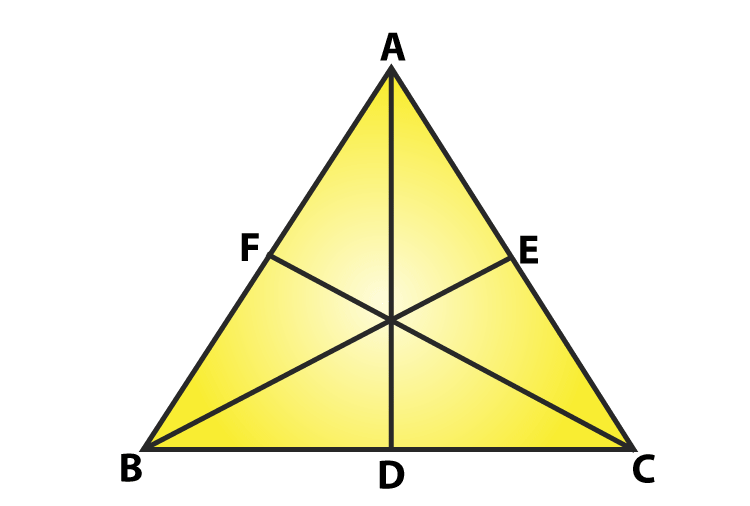Here, AD, BE and CF are medians of △ABC.

Now,

D is midpoint of BC ⇒ BD = DC

Similarly, CE = EA and AF = FB

Since ΔABC is an equilateral triangle

AB = BC = CA …..(i)

BD = DC = CE = EA = AF = FB …………(ii)

And also, ∠ ABC = ∠ BCA = ∠ CAB = 60° ……….(iii)

Consider Δ ABD and Δ BCE

AB = BC [From (i)]

BD = CE [From (ii)]

∠ ABD = ∠ BCE [From (iii)]

By SAS congruence criterion,

Δ ABD ≃ Δ BCE

[Corresponding parts of congruent triangles are equal in measure]

Now, consider Δ BCE and Δ CAF,

BC = CA [From (i)]

∠ BCE = ∠ CAF [From (iii)]

CE = AF [From (ii)]

By SAS congruence criterion,

Δ BCE ≃ Δ CAF

⇒ BE = CF …………..(v)

[Corresponding parts of congruent triangles are equal]

From (iv) and (v), we have

Median AD = Median BE = Median CF

The medians of an equilateral triangle are equal.

Hence proved

Question 5: In a Δ ABC, if ∠A = 120° and AB = AC. Find ∠B and ∠C.

Solution: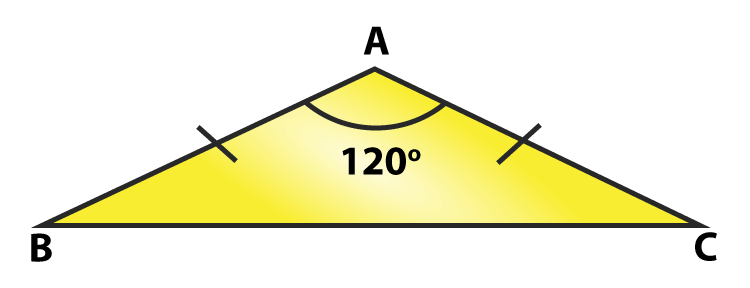To find: ∠ B and ∠ C.

Here, Δ ABC is an isosceles triangle since AB = AC

∠ B = ∠ C ……… (i)

[Angles opposite to equal sides are equal]

We know, sum of angles in a triangle = 180°

∠ A + ∠ B + ∠ C = 180°

∠ A + ∠ B + ∠ B= 180° (using (i)

1200 + 2∠B = 1800

2∠B = 1800 – 1200 = 600

∠ B = 30o

Therefore, ∠ B = ∠ C = 30

Question 6: In a Δ ABC, if AB = AC and ∠ B = 70°, find ∠ A.

Solution:

Given: In a Δ ABC, AB = AC and ∠B = 70°

∠ B = ∠ C [Angles opposite to equal sides are equal]

Therefore, ∠ B = ∠ C = 70

Sum of angles in a triangle = 180∘

∠ A + ∠ B + ∠ C = 180o

∠ A + 70o + 70o = 180o

∠ A = 180o – 140o

∠ A = 40o

## RD Sharma Solutions for Class 9 Maths Chapter 10 Congruent Triangles Exercise 10.1

RD Sharma Solutions Class 9 Maths Chapter 10 Congruent Triangles Exercise 10.1 is based on the following topics and subtopics:

• Congruence of Line segments
• Congruence of Angles
• Congruence of Triangles
• Congruence Relation
• Congruence Criteria

-SAS (Side-Angle-Side)

-SSS (Side-Side-Side)

-ASA (Angle-Side-Angle)

– RHS (Right angle- Hypotenuse-Side)

• Congruence Criterion